# PyTorch专栏（十二）：一文综述图像对抗算法

### 本文是全系列中第21 / 24篇：Pytorch 专栏【磐创AI 导读】：本篇文章讲解了PyTorch专栏的第四章中的生成对抗示例。本教程将提高您对ML（机器学习）模型的安全漏洞的认知，并将深入了解对抗性机器学习的热门话题。查看专栏历史文章，请点击下方蓝色字体进入相应链接阅读。查看关于本专栏的介绍：PyTorch专栏开篇。想要更多电子杂志的机器学习，深度学习资源，大家欢迎点击上方蓝字关注我们的公众号：磐创AI

• PyTorch简介
• PyTorch环境搭建

• 微调基于torchvision 0.3的目标检测模型
• 微调TorchVision模型
• 空间变换器网络
• 使用PyTorch进行神经传递
• 生成对抗示例
• 使用ONNX将模型转移至Caffe2和移动端

• 聊天机器人教程
• 使用字符级RNN生成名字
• 使用字符级RNN进行名字分类
• 在深度学习和NLP中使用Pytorch
• 使用Sequence2Sequence网络和注意力进行翻译### 1.威胁模型

FGSM 攻击是一种白盒攻击，其目标是错误分类。有了这些背景信息，我们现在可以详细讨论攻击。

(https://arxiv.org/abs/1412.6572)中提出，是一种简单但是有效的对抗样本生成算法。它旨在通过利用模型学习的方式和渐变来攻击神经网络。这个想法很简单，攻击调整输入数据以基于相同的反向传播梯度来最大化损失，而不是通过基于反向传播的梯度调整权重来最小化损失。换句话说，攻击是利用损失函数的梯度，然后调整输入数据以最大化损失。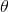代表模型参数，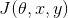是用于训练网络的损失。攻击是反向将梯度传播回输入数据以计算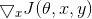### 3.实现

#### 3.1 引入相关包

from __future__ import print_functionimport torchimport torch.nn as nnimport torch.nn.functional as Fimport torch.optim as optimfrom torchvision import datasets, transformsimport numpy as npimport matplotlib.pyplot as plt

#### 3.2 输入

• epsilons：用于运行的epsilon值列表。在列表中保留0非常重要，因为它表示原始测试集上的模型性能。而且，我们期望epsilon越大，扰动就越明显，但就降低模型精度方面而言攻击越有效。由于此处的数据范围为[0,1]，因此epsilon值不应超过1。

• pretrained_model：pytorch/examples/mnist训练的预训练 MNIST模型的路径。为简单起见，请在此处下载预训练模型。

• use_cuda：如果需要和可使用CUDA的布尔标志。注意，带有CUDA的GPU对本教程并不重要，因为本教程使用CPU不会花费太多时间。

epsilons = [0, .05, .1, .15, .2, .25, .3]pretrained_model = "data/lenet_mnist_model.pth"use_cuda=True

3.3 被攻击的模型

# 定义LeNet模型class Net(nn.Module):    def __init__(self):        super(Net, self).__init__()        self.conv1 = nn.Conv2d(1, 10, kernel_size=5)        self.conv2 = nn.Conv2d(10, 20, kernel_size=5)        self.conv2_drop = nn.Dropout2d()        self.fc1 = nn.Linear(320, 50)        self.fc2 = nn.Linear(50, 10)    def forward(self, x):        x = F.relu(F.max_pool2d(self.conv1(x), 2))        x = F.relu(F.max_pool2d(self.conv2_drop(self.conv2(x)), 2))        x = x.view(-1, 320)        x = F.relu(self.fc1(x))        x = F.dropout(x, training=self.training)        x = self.fc2(x)        return F.log_softmax(x, dim=1)#声明 MNIST 测试数据集何数据加载test_loader = torch.utils.data.DataLoader(    datasets.MNIST('../data', train=False, download=True, transform=transforms.Compose([            transforms.ToTensor(),            ])),        batch_size=1, shuffle=True)# 定义我们正在使用的设备print("CUDA Available: ",torch.cuda.is_available())device = torch.device("cuda" if (use_cuda and torch.cuda.is_available()) else "cpu")# 初始化网络model = Net().to(device)# 加载已经预训练的模型model.load_state_dict(torch.load(pretrained_model, map_location='cpu'))# 在评估模式下设置模型。在这种情况下，这适用于Dropout图层model.eval()
• 输出结果：

Downloading http://yann.lecun.com/exdb/mnist/train-images-idx3-ubyte.gz to ../data/MNIST/raw/train-images-idx3-ubyte.gzExtracting ../data/MNIST/raw/train-images-idx3-ubyte.gzDownloading http://yann.lecun.com/exdb/mnist/train-labels-idx1-ubyte.gz to ../data/MNIST/raw/train-labels-idx1-ubyte.gzExtracting ../data/MNIST/raw/train-labels-idx1-ubyte.gzDownloading http://yann.lecun.com/exdb/mnist/t10k-images-idx3-ubyte.gz to ../data/MNIST/raw/t10k-images-idx3-ubyte.gzExtracting ../data/MNIST/raw/t10k-images-idx3-ubyte.gzDownloading http://yann.lecun.com/exdb/mnist/t10k-labels-idx1-ubyte.gz to ../data/MNIST/raw/t10k-labels-idx1-ubyte.gzExtracting ../data/MNIST/raw/t10k-labels-idx1-ubyte.gzProcessing...Done!CUDA Available:  True

#### 3.4 FGSM算法攻击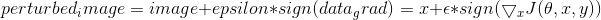# FGSM算法攻击代码def fgsm_attack(image, epsilon, data_grad):    # 收集数据梯度的元素符号    sign_data_grad = data_grad.sign()    # 通过调整输入图像的每个像素来创建扰动图像    perturbed_image = image + epsilon*sign_data_grad    # 添加剪切以维持[0,1]范围    perturbed_image = torch.clamp(perturbed_image, 0, 1)    # 返回被扰动的图像    return perturbed_image

#### 3.5 测试函数

def test( model, device, test_loader, epsilon ):    # 精度计数器    correct = 0    adv_examples = []    # 循环遍历测试集中的所有示例    for data, target in test_loader:        # 把数据和标签发送到设备        data, target = data.to(device), target.to(device)        # 设置张量的requires_grad属性，这对于攻击很关键        data.requires_grad = True        # 通过模型前向传递数据        output = model(data)        init_pred = output.max(1, keepdim=True) # get the index of the max log-probability        # 如果初始预测是错误的，不打断攻击，继续        if init_pred.item() != target.item():            continue        # 计算损失        loss = F.nll_loss(output, target)        # 将所有现有的渐变归零        model.zero_grad()        # 计算后向传递模型的梯度        loss.backward()        # 收集datagrad        data_grad = data.grad.data        # 唤醒FGSM进行攻击        perturbed_data = fgsm_attack(data, epsilon, data_grad)        # 重新分类受扰乱的图像        output = model(perturbed_data)        # 检查是否成功        final_pred = output.max(1, keepdim=True) # get the index of the max log-probability        if final_pred.item() == target.item():            correct += 1            # 保存0 epsilon示例的特例            if (epsilon == 0) and (len(adv_examples) < 5):                adv_ex = perturbed_data.squeeze().detach().cpu().numpy()                adv_examples.append( (init_pred.item(), final_pred.item(), adv_ex) )        else:            # 稍后保存一些用于可视化的示例            if len(adv_examples) < 5:                adv_ex = perturbed_data.squeeze().detach().cpu().numpy()                adv_examples.append( (init_pred.item(), final_pred.item(), adv_ex) )    # 计算这个epsilon的最终准确度    final_acc = correct/float(len(test_loader))    print("Epsilon: {}tTest Accuracy = {} / {} = {}".format(epsilon, correct, len(test_loader), final_acc))    # 返回准确性和对抗性示例    return final_acc, adv_examples

#### 3.6 运行攻击

accuracies = []examples = []# 对每个epsilon运行测试for eps in epsilons:    acc, ex = test(model, device, test_loader, eps)    accuracies.append(acc)    examples.append(ex)
• 输出结果：

Epsilon: 0      Test Accuracy = 9810 / 10000 = 0.981Epsilon: 0.05   Test Accuracy = 9426 / 10000 = 0.9426Epsilon: 0.1    Test Accuracy = 8510 / 10000 = 0.851Epsilon: 0.15   Test Accuracy = 6826 / 10000 = 0.6826Epsilon: 0.2    Test Accuracy = 4301 / 10000 = 0.4301Epsilon: 0.25   Test Accuracy = 2082 / 10000 = 0.2082Epsilon: 0.3    Test Accuracy = 869 / 10000 = 0.0869

### 4.结果

#### 4.1 准确度 vs Epsilon

plt.figure(figsize=(5,5))plt.plot(epsilons, accuracies, "*-")plt.yticks(np.arange(0, 1.1, step=0.1))plt.xticks(np.arange(0, .35, step=0.05))plt.title("Accuracy vs Epsilon")plt.xlabel("Epsilon")plt.ylabel("Accuracy")plt.show()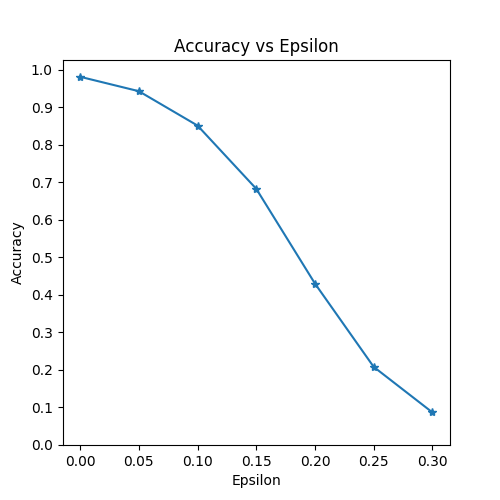#### 4.2 样本对抗性示例

# 在每个epsilon上绘制几个对抗样本的例子cnt = 0plt.figure(figsize=(8,10))for i in range(len(epsilons)):    for j in range(len(examples[i])):        cnt += 1        plt.subplot(len(epsilons),len(examples),cnt)        plt.xticks([], [])        plt.yticks([], [])        if j == 0:            plt.ylabel("Eps: {}".format(epsilons[i]), fontsize=14)        orig,adv,ex = examples[i][j]        plt.title("{} -> {}".format(orig, adv))        plt.imshow(ex, cmap="gray")plt.tight_layout()plt.show()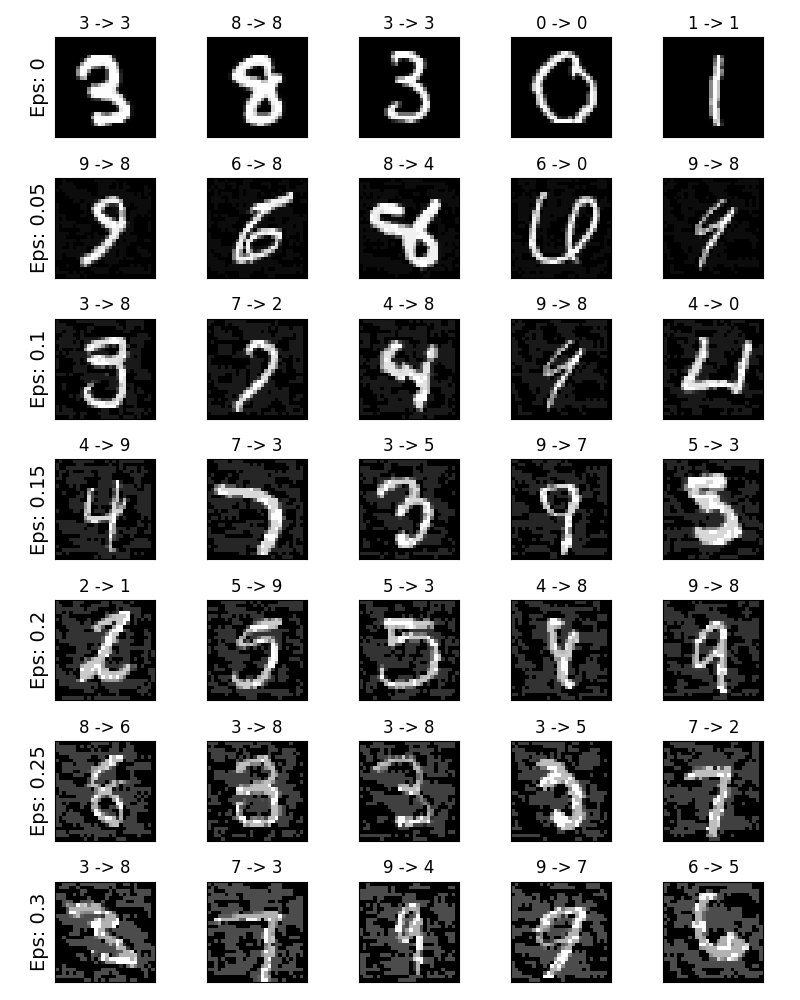### 5.展望

• [Explaining and Harnessing Adversarial  Examples]

https://arxiv.org/abs/1412.6572

• [Adversarial Attacks and Defences Competition]

https://arxiv.org/pdf/1804.00097.pdf

●  PyTorch专栏（五）：迁移学习
●  PyTorch专栏（一）
●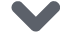下方点击 |   | 了解更多